Courses

# Chemical Potential (Part - 2)- Thermodynamic and Statistical Physics, CSIR-NET Physical Sciences Physics Notes | EduRev

## Physics for IIT JAM, UGC - NET, CSIR NET

Created by: Akhilesh Thakur

## Physics : Chemical Potential (Part - 2)- Thermodynamic and Statistical Physics, CSIR-NET Physical Sciences Physics Notes | EduRev

The document Chemical Potential (Part - 2)- Thermodynamic and Statistical Physics, CSIR-NET Physical Sciences Physics Notes | EduRev is a part of the Physics Course Physics for IIT JAM, UGC - NET, CSIR NET.
All you need of Physics at this link: Physics

Electrochemical, internal, external, and total chemical potential

The abstract definition of chemical potential given above—total change in free energy per extra mole of substance—is more specifically called total chemical potential. If two locations have different total chemical potentials for a species, some of it may be due to potentials associated with "external" force fields (Electric potential energy differences, gravitational potential energy differences, etc.), while the rest would be due to "internal" factors (density, temperature, etc.)  Therefore, the total chemical potential can be split into internal chemical potential and external chemical potential: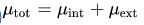where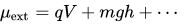i.e., the external potential is the sum of electric potential, gravitational potential, etc. (q and m are the charge and mass of the species, respectively, V and h are the voltage and height of the container, respectively, and g is the acceleration due to gravity.) The internal chemical potential includes everything else besides the external potentials, such as density, temperature, and enthalpy.

The phrase "chemical potential" sometimes means "total chemical potential", but that is not universal. In some fields, in particular electrochemistry, semiconductor physics, and solid-state physics, the term "chemical potential" means internal chemical potential, while the term electrochemical potential is used to mean total chemical potential.

Systems of particles

Chemical potential of electrons

Electrons in solids have a chemical potential, defined the same way as the chemical potential of a chemical species: The change in free energy when electrons are added or removed from the system. In the case of electrons, the chemical potential is usually expressed in energy per particle rather than energy per mole, and the energy per particle is conventionally given in units of electronvolt (eV).

Chemical potential plays an especially important role in solid-state physics and is closely related to the concepts of work function, Fermi energy, and Fermi level. For example, n-typesilicon has a higher internal chemical potential of electrons than p-type silicon. In a p–n junction diode at equilibrium the chemical potential (internal chemical potential) varies from the p-type to the n-type side, while the total chemical potential (electrochemical potential, or, Fermi level) is constant throughout the diode.

As described above, when describing chemical potential, one has to say "relative to what". In the case of electrons in semiconductors, internal chemical potential is often specified relative to some convenient point in the band structure, e.g., to the bottom of the conduction band. It may also be specified "relative to vacuum", to yield a quantity known as work function, however work function varies from surface to surface even on a completely homogeneous material. Total chemical potential on the other hand is usually specified relative to electrical ground.

In atomic physics, the chemical potential of the electrons in an atom is sometimes  said to be the negative of the atom's electronegativity. Likewise, the process of chemical potential equalization is sometimes referred to as the process of electronegativity equalization. This connection comes from the Mulliken electronegativity scale. By inserting the energetic definitions of the ionization potential and electron affinity into the Mulliken electronegativity, it is seen that the Mulliken chemical potential is a finite difference approximation of the electronic energy with respect to the number of electrons., i.e.,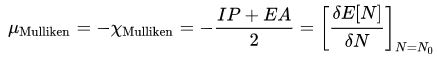where IP and EA are the ionization potential and electron affinity of the atom, respectively.

Sub-nuclear particles

In recent years, thermal physics has applied the definition of chemical potential to systems in particle physics and its associated processes. For example, in a quark–gluon plasma or other QCD matter, at every point in space there is a chemical potential for photons, a chemical potential for electrons, a chemical potential for baryon number, electric charge, and so forth.

In the case of photons, photons are bosons and can very easily and rapidly appear or disappear. Therefore, the chemical potential of photons is always and everywhere zero. The reason is, if the chemical potential somewhere was higher than zero, photons would spontaneously disappear from that area until the chemical potential went back to zero; likewise if the chemical potential somewhere was less than zero, photons would spontaneously appear until the chemical potential went back to zero. Since this process occurs extremely rapidly (at least, it occurs rapidly in the presence of dense charged matter), it is safe to assume that the photon chemical potential is never different from zero.

Electric charge is different, because it is conserved, i.e. it can be neither created nor destroyed. It can, however, diffuse. The "chemical potential of electric charge" controls this diffusion: Electric charge, like anything else, will tend to diffuse from areas of higher chemical potential to areas of lower chemical potential. Other conserved quantities like baryon number are the same. In fact, each conserved quantity is associated with a chemical potential and a corresponding tendency to diffuse to equalize it out.

In the case of electrons, the behavior depends on temperature and context. At low temperatures, with no positrons present, electrons cannot be created or destroyed. Therefore, there is an electron chemical potential that might vary in space, causing diffusion. At very high temperatures, however, electrons and positrons can spontaneously appear out of the vacuum (pair production), so the chemical potential of electrons by themselves becomes a less useful quantity than the chemical potential of the conserved quantities like (electrons minus positrons).

The chemical potentials of bosons and fermions is related to the number of particles and the temperature by Bose–Einstein statistics and Fermi–Dirac statistics respectively.

Chemical Potential in Ideal vs. Non-Ideal Solutions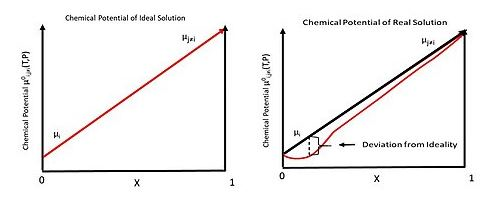The chemical potential of component i in solution for (left) ideal and (right) real solutions.

In an ideal solution, the chemical potential of species i (μi) is dependent on temperature and pressure. μi0(T,P) is defined as the chemical potential of pure species i. Given this definition the chemical potential of species i in an ideal solution is: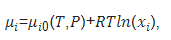where R is the gas constant and xi is the mole fraction of species i contained in the solution.

This equation assumes that μi only depends on the mole fraction (xi) contained in the solution. This neglects intermolecular interaction between species i with itself and other species [i-(j≠i)]. This can be corrected for by factoring in the coefficient of activity of species i, defined as γi. This correction yields: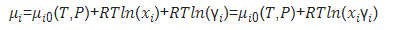The images at the above give a rough picture of the ideal and non-ideal situation.

Offer running on EduRev: Apply code STAYHOME200 to get INR 200 off on our premium plan EduRev Infinity!

159 docs

,

,

,

,

,

,

,

,

,

,

,

,

,

,

,

,

,

,

,

,

,

,

,

,

;